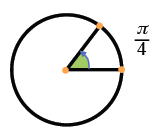### Home > PC > Chapter 3 > Lesson 3.1.3 > Problem3-47

3-47.

Sketch a graph of the unit circle then draw and label the following angles.

1.  $\frac{\pi}{4}$1.  $- \frac { \pi } { 3 }$

Wrap clockwise from $\left(1, 0\right)$ because of the $−$ sign.

1.  $3 \pi$

Wrap counter-clockwise more than once. How much more?